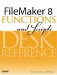# Mod()

Category: Number

 Syntax: Mod ( number; divisor )

Parameters:

numberAny expression that resolves to a numeric value.

divisorAny expression that resolves to a numeric value.

Data type returned: Number

Description:

Returns the remainder after number is divided by divisor.

Mod() is related to the Div() function; Div() returns the whole number portion of x divided by y, whereas Mod() returns the remainder.

There are many practical uses for the Mod() function. For instance, when x is an integer, Mod (x; 2) returns 0 if x is even, and 1 if x is odd. Mod (x; 1) returns just the decimal portion of a number.

Examples:

Function

Results

Mod (7; 5)

Returns 2.

Mod (-7; 5)

Returns 3.

Mod (13; 3)

Returns 1.

Mod (1.43; 1)

Returns .43.FileMaker 8 Functions and Scripts Desk Reference
ISBN: 0789735113
EAN: 2147483647
Year: 2004
Pages: 352